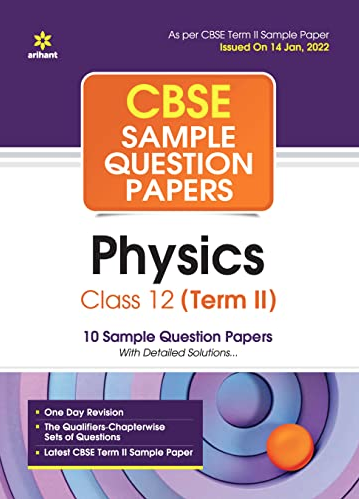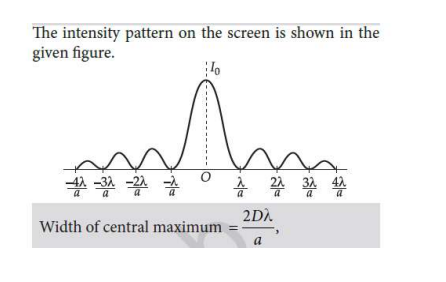# (a) “If the slits in Young’s double slit experiment are identical, then intensity at any point on the screen may vary between zero and four times to the intensity due to single slit”. Justify the above statement through a relevant mathematical expression. (b) Draw the intensity distribution as function of phase angle when diffraction of light takes place through coherently illuminated single slit.

CBSE Sample Question Paper, Class 12 Physics Term 2 Question - (a) "If the slits in Young's double slit experiment are identical, then intensity at any point on the screen may vary between zero and four times to the intensity due to single slit". Justify the above statement through a relevant mathematical expression. (b) Draw the intensity distribution as function of phase angle when diffraction of light takes place through coherently illuminated single slit.## (a) “If the slits in Young’s double slit experiment are identical, then intensity at any point on the screen may vary between zero and four times to the intensity due to single slit”. Justify the above statement through a relevant mathematical expression. (b) Draw the intensity distribution as function of phase angle when diffraction of light takes place through coherently illuminated single slit.

### ANS. 11 (b) Intensity distribution as function of phase angle, when diffraction of light takes place through coherently illuminated single slit